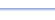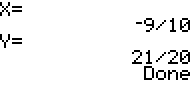Program: CRAMER

CRAMER

• Solves systems of linear equations in two or three variables.  (The program does this by a method known as Cramer's Rule, which is why it is called 'CRAMER'.)

Useful for:

• SAT
• ACT
• Algebra 1
• Geometry
• Algebra 2
• Precalculus
• Calculus
• College Algebra

See full list of programs

Try these problems using CRAMER:
on MathPro Q&A ForumExample 1

Q: Solve the system of equations:
8x + 4y = -3
2y - x - 3 =0

Solution:  First, put both equations in standard form:
8x + 4y = -3
-1x + 2y = 3

Run CRAMER.  How?
Enter '2' for two
variables (x and y).

Enter the coefficients
shown in red above,
from left to right, for
A, B, C, D, E, and F.

The program reports:

Interpretation:  x = -9/10 and y = 21/20

See Example 2